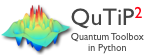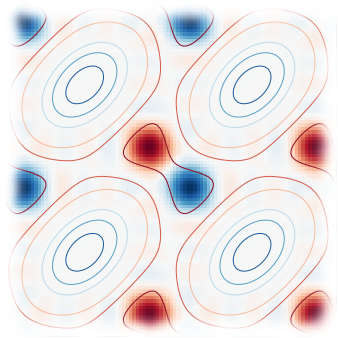# Scientific computing projectsQuTiP is an open-source python framework for simulating the dynamics of quantum systems. QuTiP is based on the excellent Numpy and Scipy numerical packages, and graphical output is provided by Matplotlib. QuTiP aims to provide user-friendly and efficient numerical simulations of a wide variety of quantum mechanical problems commonly found in physics applications in fields such as quantum optics, trapped ions, superconducting circuits, and quantum nanomechanical resonators.

More information and documentation is available at the QuTiP web page, and at the QuTiP github repositories.wavefunction is a Python package for calculating wavefunctions, energy levels, transition rates, etc., for 1 and 2 dimensional potentials. It includes examples for the harmonic oscillator, flux and current bias phase qubits, the Morse potential, and Flux qubits.

#### IPython extensions

Various extensions for use with the IPython notebook.

#### Lectures on QuTiP

A series of computationally-oriented lectures on quantum mechanics and quantum optics using QuTiP and iPython notebooks. The notebooks can be downloaded from the qutip-lectures github repository (ZIP), or viewed online (read-only) using the links below. The following topics are covered:

#### Symbolic quantum mechanics

IPython notebooks on symbolic quantum mechanics with SymPy and SymPsi. The notebooks are available from the sympsi-notebooks repository at github, and can be view as static HTML pages via these links:

#### Wavefunction notebooks

IPython notebooks with examples for the wavefunction python package. More examples are available in the github repository.

#### Lectures on scientific computing with Python

A series of lectures on scientific computing using python. The lectures are written as IPython notebooks, which means that you can interactively edit and run the code. Download the notebooks from the scientific-python-lectures github repository (ZIP), or browse them online (read-only) using the these links:

#### Reproduced papers

A collection of IPython notebooks with reproduced numerical results (to varying degree of detail) for some interesting papers. The notebooks are available for download at the reproduced-papers github repository, and can be previewed online using the following links: